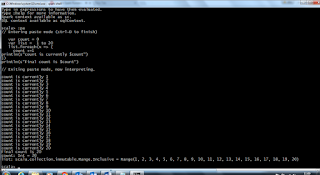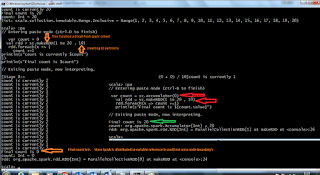Sunday, July 17, 2016

Spark Closures, Broadcasting , Optimizing and Partitioning

This post will explain you about how to do Optimization in Spark and how to work with closures, Broadcasting and partitioning.

1. Closures
- It is standalone function, which contains at least one bound variable
var count = 0
var list =  1 to 20
list.foreach(x => {
count +=1
println(s"count is currently \$count")
})
println(s"Final count is \$count")How to use Closures in our Spark?
1. Since Spark distributed so variable reference is could not cross node boundary’s.
So each partition will get it’s own copy of variables.
var count = 0
val rdd = sc.makeRDD(1 to 20 , 10)
rdd.foreach(x => {
count +=1
println(s"count is currently \$count")
})
println(s"Final count is \$count")2. This happens in outside Driver . So final count will not be updated.
3. For this we will us built in methods

val indexer =Map(…) //1MB - it will be distributed across clusters for each execution
rdd.flatMap(rddVal => indexer.get(rddVal))
a. Usually Map will distribute Simple 1MB data into multiple workers and store size will be 10 to 11 MB data
b. To avoid this we have broadcast variables into place
val indexer = sc.brodcast(Map(…)) //Map 1MB ; indexer<1MB rdd.flatMap(rddVal = >indexer.value.get(rddVal))
3. Optimizing Partitioning
a. Make RDD with lot of data with 10000 chunks
b. Then use the filter to drastically reduces the data set
c. Then we will do the some more transformations before calling the final collect.
sc.makeRDD(1 to Int.MaxValue,10000).filter(x=>x < 10).sortBy(x=>x).map(x=>x+1).collect
sc.makeRDD(1 to Int.MaxValue,10000).filter(x=>x < 10).coalesce(8,true).sortBy(x=>x).map(x=>x+1).collect

We can check the jobs data using http://localhost:4040How normal partition will work as how partition will work with coalesceThis is how spark advanced concepts will work.
Thank you very much for viewing this post.